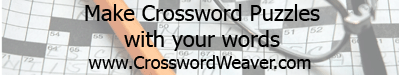At the Vending Machine

I  S  P  E  P  M  P  D  Z  C  M  O  A

F  B  R  K  Z  R  R  U  F  B  A  T  L

A  D  L  E  P  O  O  P  N  V  Q  G  O

N  M  I  A  P  U  R  O  I  E  K  V  C

T  P  E  A  C  P  Z  A  T  B  V  D  C

A  E  O  T  M  K  E  A  N  B  B  E  R

S  P  Q  R  I  E  C  P  T  G  E  W  S

H  S  U  B  A  R  T  H  R  S  E  E  A

C  I  T  C  S  N  P  U  E  D  A  R  R

L  O  J  O  L  T  G  S  N  R  T  H  R

E  N  Y  K  Y  Y  T  E  M  I  R  J  S

W  E  A  E  P  A  R  G  C  J  M  Y  E

C  O  K  E  C  L  A  S  S  I  C  T  R

 Black Cherry Jolt RC Cola Citra Minute Maid Rootbeer Coke Mr Pibb Seven Up Coke Classic Orange Shasta Dr Pepper Orange Sprite Fanta Pepsi Tab Grape Pepsi One Welchs